•#### Quantum Machine Learning and Optimisation in Finance#### Overview of this book

With recent advances in quantum computing technology, we finally reached the era of Noisy Intermediate-Scale Quantum (NISQ) computing. NISQ-era quantum computers are powerful enough to test quantum computing algorithms and solve hard real-world problems faster than classical hardware. Speedup is so important in financial applications, ranging from analysing huge amounts of customer data to high frequency trading. This is where quantum computing can give you the edge. Quantum Machine Learning and Optimisation in Finance shows you how to create hybrid quantum-classical machine learning and optimisation models that can harness the power of NISQ hardware. This book will take you through the real-world productive applications of quantum computing. The book explores the main quantum computing algorithms implementable on existing NISQ devices and highlights a range of financial applications that can benefit from this new quantum computing paradigm. This book will help you be one of the first in the finance industry to use quantum machine learning models to solve classically hard real-world problems. We may have moved past the point of quantum computing supremacy, but our quest for establishing quantum computing advantage has just begun!
PrefaceFree Chapter
Chapter 1: The Principles of Quantum MechanicsPart I: Analog Quantum Computing – Quantum AnnealingPart II: Gate Model Quantum Computing# 1.1 Linear Algebra for Quantum Mechanics

Quantum computing and quantum mechanics rely on a specific notational formalism, due to Dirac, and are supported by classical linear algebra, in particular Hermitian structures of matrices and tensor products. We provide here a self-contained review of these tools to facilitate the understanding of the rest of the book. We start with basic linear algebra principles before introducing Dirac notations and the quantum counterparts of linear algebra tools. Sections 1.1.1 to 1.1.4 concentrate on standard definitions of finite-dimensional Hilbert spaces and matrices, while Sections 1.1.5 to 1.1.7 review the key details and properties of complex matrices (decompositions, Hermitian property, and rotations). Sections 1.1.9 to 1.1.11 introduce Dirac’s formalism and the essential aspects of quantum operators.

## 1.1.1 Basic definitions and notations

We let 𝔽 denote either the real field or the complex one . For a complex number z = x+iy , with x,y , we write the conjugate z := xiy. We let m,n(𝔽) denote the space of matrices of dimension m × n with entries in 𝔽 and n(𝔽) whenever m = n. For A := (aij)1im; 1jn ∈ℳm,n(𝔽), A := (aij)1im; 1jn is the complex conjugate. If A ∈ℳn(𝔽), we write A for its transpose and A := (A) for its Hermitian conjugate. We finally denote I the identity matrix and write In whenever we wish to emphasise the dimension, and 0m,n the null matrix in m,n(𝔽). Recall that a matrix A ∈ℳn(𝔽) is invertible (or non-singular) if there exists B ∈ℳn(𝔽) such that AB = BA = In. Given two matrices A ∈ℳp,m(𝔽) and B ∈ℳq,n(𝔽), we define their tensor product as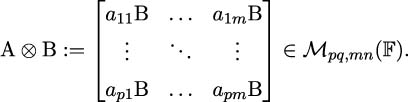Since a vector is a particular case of a matrix, for u 𝔽m and v 𝔽n, we can write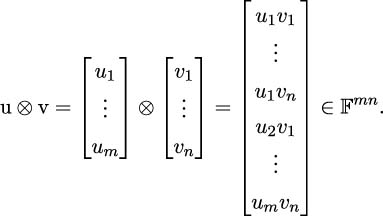## 1.1.2 Inner products

A vector space V over the field 𝔽 is a set endowed with

• a commutative, associative addition operation,
• an operation of multiplication by a scalar.

The addition and the multiplication by a scalar have the following properties (for scalars α,β 𝔽 and vectors u,v V):

• v + 0 = v;
• v + (v) = 0;
• α(βv) = (αβ)v;
• (α + β)v = αv + βv;
• α(u + v) = αu + αv;
• 1 v = v.

Armed with this, we can now define an inner product on V:

Definition 1. A map ⟨⋅,⋅⟩ : V ×V 𝔽 is called an inner product if, for u,v,w V and α 𝔽,

• (Positive definiteness) u,u⟩≥ 0 and u,u= 0 if and only if u = 0;
• (Conjugate symmetry) u,v= v,u;
• (Linear in the first argument) u + v,w= u,w+ v,wand αu,v= αu,v;
• (Antilinear in the second argument) u,v + w= u,v+ u,wand uv= αu,v.

The inner product is further called non-degenerate if u,v= 0 for all v V ∖{0} implies u = 0.

For example, the following spaces carry a natural inner product:

• The vector space n with the inner product u,v:= uv = i=1nuivi;
• The space of complex-valued continuous functions on [0,1] with f,g:= 01f(t)g(t)dt;
• If X,Y ∈ℳm,n(), then X,Y:= Tr(XY) = i=1m j=1nXijYij defines an inner product on the space of (real) matrices.

Projection matrices are particularly useful for geometric purposes:

Definition 2. A matrix P ∈ℳn(𝔽) is called a (orthogonal) projection if P2 = P.

In particular, if W is a vector subspace of 𝔽n with some orthonormal basis (w1,…,wd), it is then easy to check that the map 𝒫W : 𝔽n 𝔽n onto W satisfying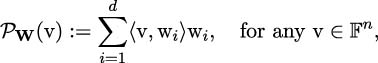defines an orthogonal projection.

## 1.1.3 From linear operators to matrices

Let V be a finite-dimensional vector space over 𝔽 and ⟨⋅,⋅⟩ a non-degenerate inner product on V. Given a linear operator 𝒜 : V V, then, by the Riesz representation theorem  [309, Section III-6], there exists a unique linear operator 𝒜 : V V, called the adjoint operator, such that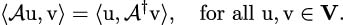Indeed, for any v V, the map u V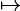⟨𝒜u,vis a linear functional, hence an element of the dual space V (the space of bounded linear functionals on V), therefore for each v V, there exists v′∈V such that ⟨𝒜u,v= u,v′⟩. It is then easy to show that the map v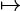vis linear, proving that the adjoint operator is uniquely defined. In the particular case where 𝒜 = 𝒜, the operator 𝒜 is called Hermitian, a key requirement in quantum mechanics:

Definition 3. The operator 𝒜 is called Hermitian, or self-adjoint, if 𝒜 = 𝒜.

For a Hermitian operator 𝒜, we then have, for any u V,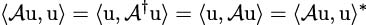by conjugate symmetry (Definition 1), and therefore ⟨𝒜u,uis real. Conversely, if ⟨𝒜u,uis real, then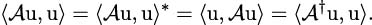Therefore,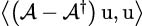= 0; since this is true for all u V, then 𝒜 = 𝒜.

The following property of operators shall be useful to ensure that systems driven by operators preserve distances, or norms:

Definition 4. The linear operator 𝒜 : V V is called unitary if it is surjective and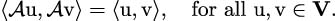Recall that a linear operator between two finite-dimensional normed spaces is bounded, and therefore continuous. For any u V, this implies that ∥𝒜u= u, so that a unitary operator 𝒜 preserves the norm. In that case, 𝒜 is an isometry, therefore injective. Being also surjective, it is bijective and therefore its inverse exists. For a unitary operator 𝒜 and any u,v V, we have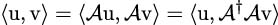by definition of the adjoint, implying that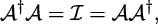where is the identity operator.

Example (Real Matrices): If V = n with inner product u,v:= uv for u,v n, the linear operator 𝒜 can now be viewed as a matrix A in n(). Its adjoint is nothing other than the transpose A, and therefore A is self-adjoint if and only if it is symmetric. In this case, if A is unitary (or orthogonal), then it is invertible with A1 = A. Rotation matrices in 2, which will play an important role later when constructing quantum circuits, are the only unitary maps of 2 onto itself and are of the form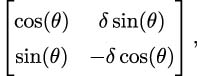for 𝜃 [0,2π) and δ ∈{−1,+1}.

Example (Complex Matrices): If V = n with inner product u,v:= vu for u,v n, the linear operator 𝒜 can now be viewed as a matrix in n(). The adjoint of such a matrix is then the Hermitian conjugate A and A is called Hermitian if A = A and unitary if AA = In. We shall denote by 𝒰n() the set of unitary matrices in n(). We will discuss Hermitian matrices over in more detail in Section 1.1.6.

## 1.1.4 Condition number

In order to manipulate matrices and measure them, we require matrix norms:

Definition 5. A matrix norm ∥⋅∥ : m,n(𝔽) is a function satisfying, for any α 𝔽 and A,B ∈ℳm,n(𝔽),

• (positively valued) A∥≥ 0;
• (definite) A= 0 if and only if A = 0m,n;
• (absolutely homogeneous) αA= |α|∥A;
• (triangle inequality) A + B∥≤∥A+ B.

The norm is further called sub-multiplicative if AB∥≤∥A∥∥B.

The condition number of a matrix is an important tool to understand the stability of linear equations of the form Ax = b, for A ∈ℳn(𝔽), b 𝔽n. Assuming A to be non-singular, the true solution is clearly x := A1b. Suppose, however, that the vector b is only known up to some (not necessarily quantum) measurement error, and one observes instead b + Δb. The solution is then A1(b + Δb) = x + Δx, with Δx := A1Δb. In particular, we can write, for any (sub-multiplicative) matrix norm ∥⋅∥,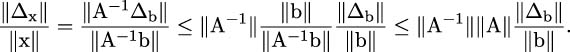From this inequality, we see that the quantity A1∥∥Abounds the relative error in the solution with respect to the relative error in the measurement of the input vector b. This leads to the following terminology:

Definition 6. Given a matrix A ∈ ℳn(𝔽) and a sub-multiplicative norm ∥⋅∥, we call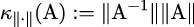the condition number (with respect to the norm ∥⋅∥) of the matrix A (and assign to it infinite value if A is singular).

Remark: The definition of the condition number above holds for any matrix norm ∥⋅∥, but admits a more explicit representation in the particular case of the spectral norm ∥⋅∥2, defined as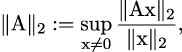where x2 :=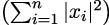is the L 2 norm for vectors. If the matrix A is not singular, then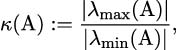where λmax(A) and λmin(A) denote the largest and smallest eigenvalues of A.

## 1.1.5 Matrix decompositions and spectral theorem

Having defined essential properties of (complex) matrices, we now introduce several essential tools that allow us to gain a better understanding of their properties.

The Singular Value Decomposition is a key tool to analyse the properties and behaviours of matrices. It is ubiquitous in applied statistics and machine learning and allows us to reduce the explanatory dimension of a large matrix into a small number of meaningful components.

Theorem 1 (Singular Value Decomposition). Let A ∈ℳm,n(𝔽) and p := min(m,n). There exist U ∈𝒰m(𝔽), V ∈𝒰n(𝔽) and σ1σp 0 such that A = UΣV, where Σ ∈ℳm,n(𝔽) is diagonal with Σii = σi for i = 1,…,p and Σii = 0 for i > p.

The numbers {σ1,…,σp} are called the singular values of A and are uniquely defined. The columns of U and V are the left-singular and right-singular vectors of A, in the sense that, if σ ∈{σ1,…,σp}, then there exist a column u of U and a column v of V such that Av = σu and Au = σv. Recall that the rank of a matrix is defined as the dimension of the span of its columns. As a corollary of the Singular Value Decomposition theorem, the rank of a matrix is therefore equal to the number of non-zero singular values. The Singular Value Decomposition is general in the sense that it holds for any matrix. In the particular case of square matrices, the Schur decomposition and the Spectral Theorem provide refinements.

The Spectral Theorem is a cornerstone result in the theory of linear operators, and in particular for (finite-dimensional) matrices. Recall that an operator 𝒜 : V V is called normal if it commutes with its adjoint, namely if 𝒜𝒜 = 𝒜𝒜. Self-adjoint (or Hermitian) operators are clearly normal, yet the converse is not true in general. Recall further that an eigenvector of 𝒜 is a non-zero vector u V such that 𝒜u = λu for some λ , and we denote by σ(𝒜) the set of eigenvalues of 𝒜.

The following result, which is more general than the subsequent spectral theorem, allows us to decompose any arbitrary complex square matrix.

Theorem 2 (Schur Decomposition). For any A ∈ ℳn() there exits a unitary matrix U ∈ 𝒰n() and an upper triangular matrix T such that A = UTU1.

Note that since U is unitary, then U1 = U. We call the matrix T the Schur transform of A and the identity in the theorem means that A and T are similar, so in particular, possess the same eigenvalues, all located on the diagonal of T. If A is a normal matrix, then so is T, and therefore T must be diagonal and we write T = D for clarity. In this case, we say that the matrix A is diagonalisable with A = UDU, where the diagonal entries of D are the eigenvalues of A and the column vectors of U are the orthonormal eigenvectors of A.

Theorem 3 (Spectral Theorem). The linear operator 𝒜 : V V is normal if and only if there exists an orthonormal basis of V consisting of eigenvectors of A.

For each eigenvalue λ σ(𝒜), denote the corresponding eigenspace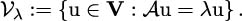Since the vector space V is the orthogonal direct sum of the eigenspaces (indexed by the eigenvalues of 𝒜), we can then write the spectral decomposition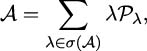where 𝒫λ is the orthogonal projection operator onto 𝒱λ. Note that such an operator is naturally self-adjoint  [309, Theorem 2, Section III-1].

## 1.1.6 Hermitian matrices

We introduced above Hermitian matrices as the set of matrices A over the complex field such that A = A. As fundamental building blocks of quantum computing, we investigate their properties further. Clearly, a real matrix is Hermitian if and only if it is symmetric, in which case A = A.

Proposition 1. The eigenvalues of a Hermitian matrix are real.

Proof. If Ax = λx for λ and x n, then

 ⟨Ax,x⟩ = x†Ax = λx†x = λ∥x∥2, ⟨x,Ax⟩ = (Ax)†x = (λx)†x = λ∗x†x = λ∗∥x∥2.

Since both are equal by the Hermitian property, then λ = λ, proving the proposition. □

The Singular Value Decomposition (Theorem 1) takes a particular flavour in the case of Hermitian matrices:

Theorem 4. With the notations of Theorem 1, if A ∈ ℳn() is Hermitian, then the matrices U and V are equal and the matrix Σ is diagonal with real entries.

Theorem 5. For a Hermitian matrix A ∈ ℳn(), the following are equivalent:

1. The eigenvalues are non-negative.
2. There exists a Hermitian matrix B ∈ℳn() such that A = B2.
3. There exists a matrix B ∈ℳn() such that A = BB.
4. For every x n, Ax,x⟩≥ 0.

Such a matrix is called positive semi-definite.

Proof. The Spectral Theorem shows that there exist a unitary matrix U ∈𝒰n() and a diagonal matrix Σ ∈ℳn() such that A = UΣU, where the diagonal elements of Σ are the eigenvalues of A. Assuming (i), we can define B = U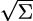U∈ℳn(). Then clearly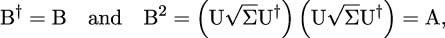since U is unitary. The equality A = BB is also obvious. The latter implies that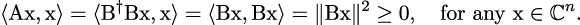Finally, assume (iv) and let λ be an eigenvalue of A with eigenvector u. Then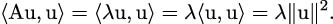Since the latter is strictly positive, then clearly λ 0.

The following property lies at the core of Hamiltonian simulation of quantum systems:

Theorem 6. If A ∈ ℳn() is Hermitian, then, for any t , eitA is unitary; conversely, every unitary matrix has the form eitA for some Hermitian matrix A.

Recall that for a matrix A ∈ℳn(), its exponential is given by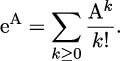In practice, though, given a Hermitian matrix A, finding the corresponding unitary matrix U is not easy. The Hamiltonian simulation problem is defined as follows.

Hamiltonian Problem: Given a Hermitian matrix A ∈ℳn(), a time t > 0, a tolerance level 𝜀 > 0, and some matrix norm ∥⋅∥, find a unitary matrix U such that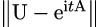𝜀.

## 1.1.7 Rotation matrices

Rotation matrices, and later their quantum gate equivalents will play a key role in building quantum circuits. Let us start with the following lemma:

Lemma 1. If a matrix A ∈ℳn() is such that A2 = I, then for any 𝜃 ,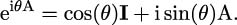Proof. This follows directly from the series expansion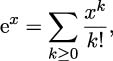which has an infinite radius of convergence. □

Lemma 1 will prove essential for computational purposes. As simple examples, consider the following:

Exercise: Compute ei𝜃A for A ∈{X,Y,Z} and 𝜃 , where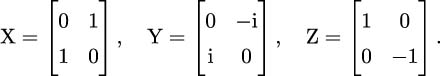For any α [0,2π), consider now the map α : 2 2 such that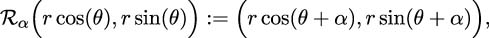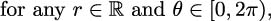which is basically a rotation of angle α and does not affect the norm of the input vector. To the map α, we can associate the (rotation) matrix Rα such that α(u) = Rαu for any u 2. It is easy (exercise) to show the following:

Lemma 2. The matrix Rα has the form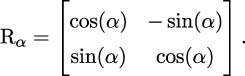This representation is the general form of a rotation matrix in 2 (introduced in (1.1.3)).

Exercise: Write the matrices ei𝜃A for A ∈{X,Y,Z} from the previous exercise as rotation matrices.

## 1.1.8 Polar coordinates

Recall that a point z = x + iy, with x,y , lying on the unit circle can be written as z = ei𝜃 with 𝜃 [0,2π). Indeed, simply let x = r cos(𝜃), y = r sin(𝜃) and add the constraint r = 1. Consider now a general vector u 2 of the form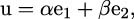with α,β such that |α|2 + |β|2 = 1. Here, (e1,e2) forms a basis of 2: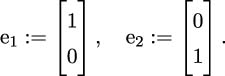In polar coordinates, we can then write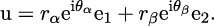Note that arbitrary multiplication phases have no influence – a fact of key importance in quantum mechanics – because, for any γ ,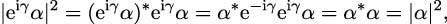so that in fact, multiplying u by the global phase ei𝜃α and letting 𝜃 := 𝜃β 𝜃α, we consider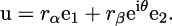Write temporarily rβei𝜃 = x + iy. Insisting on u being on the unit sphere further imposes u2 = 1, namely

 1 = ∥u∥2 =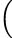rαe1 + (x + iy)e2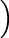†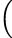rαe1 + (x + iy)e2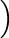=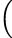rαe1⊤ + (x − iy)e 2⊤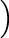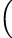rαe1 + (x + iy)e2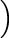= rα2 + x2 + y2,

since (e1,e2) is orthonormal. This is nothing more than the equation of the unit sphere. In polar coordinates, we can write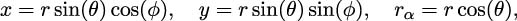and clearly r = 1 since we are on the unit sphere. Therefore

 u = cos(𝜃)e1 +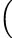sin(𝜃)cos(ϕ) + isin(𝜃)sin(ϕ)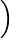e2 = cos(𝜃)e1 + sin(𝜃)eiϕe 2.

## 1.1.9 Dirac notations

Given a vector v n, Dirac’s ket and bra notations read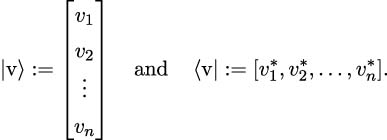With these notations, the operation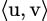:= u|vdefines an inner product on n. The notation for the standard orthonormal basis in  n is (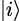)i=0,…,n1, i.e.,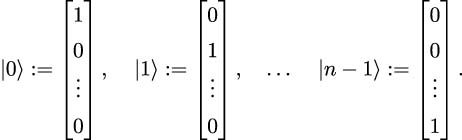In coordinates, we can write, for any u,v n,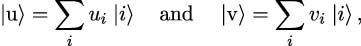and therefore,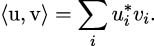## 1.1.10 Quantum operators

In the language of Dirac’s notations, we can define the outer product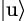v| (for u U and v V) as a linear operator from V to U, two vector spaces, as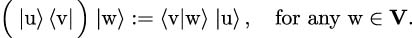In particular,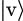v| is the projection on the one-dimensional space generated by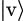. Any linear operator can be expressed as a linear combination of outer products as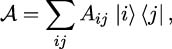where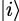and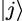are the standard basis vectors (1.1.9).

Similarly to the linear algebra setting above, we can define an eigenvector of a linear operator 𝒜 : V V as a non-zero vector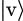such that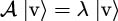for some complex eigenvalue λ. Associated with any linear operator 𝒜, the adjoint operator 𝒜 satisfies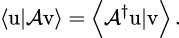Indeed, in the language of linear operators above, we have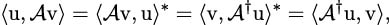by definition of the inner product (Definition 1).

## 1.1.11 Tensor product

Given two vector spaces U and V of dimensions m and n, the tensor product U V is a vector space of dimension mn. For u U and v V, we can form the vector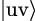:=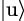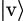U V with the following properties:

•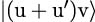=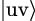+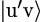, for any u′∈U;
•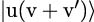=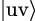+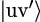, for any v′∈V;
• α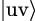=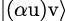=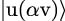, for any α .

Given the linear operators 𝒜 : U U and : V V, we can then define their tensor product as an operator 𝒜⊗ℬ on U V: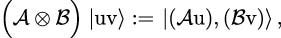which can be represented in matrix form as A B ∈ℳmn,mn().

This Dirac formalism, fully anchored in (classical) linear algebra, now opens the gates to a proper dive into the foundations of quantum mechanics.